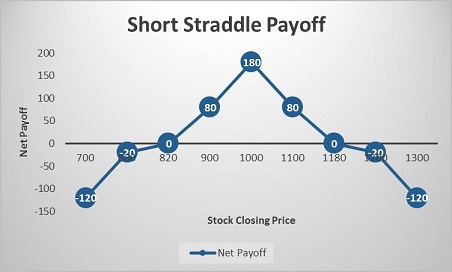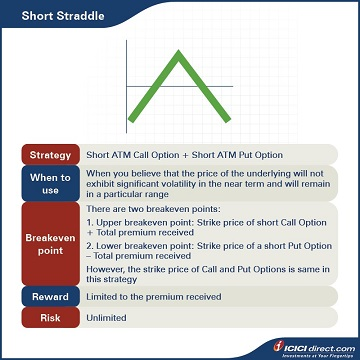Abhinav’s manager, Simran, tells him that her market outlook for stock ABC Ltd is rangebound in the near term. Accordingly, she asks Abhinav to suggest an Options strategy for ABC Ltd. Abhinav, after careful consideration, suggests the Short Straddle strategy. Let’s take a look at the details of this strategy.

In a Short Straddle, the trader sells a Call and a Put on the same underlying for the same expiry and strike price. The Options sold are normally ATM Options. The risk in the Short Straddle is unlimited. However, returns are capped to the extent of premium received.

Strategy: Short ATM Call Option (Leg 1) + Short ATM Put Option (Leg 2)

When to use: When you believe that the price of the underlying will not exhibit significant volatility in the near term, and will remain in a particular range

Breakeven: There are two breakeven points:

1. Upper breakeven point: Strike price of short Call Option + Total premium received

2. Lower breakeven point: Strike price of a short Put Option – Total premium received

However, the strike price of the Call and Put Options is the same in this strategy.

Maximum risk: Unlimited

Let’s understand this strategy with an example:

Abhinav sells ABC Ltd. ATM Call and Put Options at a strike price of Rs. 1,000, with premiums of Rs. 100 and Rs. 80 respectively. Abhinav receives a total premium of Rs. 100 + Rs. 80 = Rs. 180, and this will be the maximum profit. He will lose money if the stock moves more than 180 points in any direction i.e., above Rs. 1,180 or below Rs. 820.

### Let’s look at the cash flow in various scenarios:

 Closing price of ABC Ltd. on expiry (Rs.) Payoff from ATM Call Option (A) (Rs.) Payoff from ATM Put Option (B) (Rs.) Net payoff (A+B) (Rs.) 700 100 – 220 – 120 800 100 – 120 – 20 820 100 – 100 0 900 100 – 20 80 1000 100 80 180 1100 0 80 80 1180 –80 80 0 1200 –100 80 – 20 1300 –200 80 – 120

Let us understand the payoff in various scenarios. It will give you a fair idea of how we have arrived at the above values.

### If the stock closes at Rs. 800 on expiry: Leg 1 expires OTM while leg 2 expires ITM

Leg 1: Premium received on the ATM Call Option of strike price Rs. 1000 = Rs. 100

Premium paid on ATM Call Option of strike price Rs. 1000 at expiry = Max {0, (Spot price – Strike price)} = Max {0, (800 – 1000)} = Max (0, – 200) = 0

So, the payoff from the ITM Call Option = Premium received – Premium paid = 100 – 0 = Rs. 100

Leg 2: Premium received on the ATM Put Option of strike price Rs.1000 = Rs. 80

Premium paid on ATM Put Option of strike price Rs. 1000 at expiry = Max {0, (Strike price – Spot price)} = Max {0, (1000 – 800)} = Max (0, 200) = Rs. 200

So, the Payoff from the ATM Put Option = Premium received – Premium paid = 80 – 200 = – Rs. 120

Net payoff = Payoff from ATM Call Option + Payoff from ATM Put Option = 100 + (– 120) = – Rs. 20

### If the stock closes at Rs. 1000 on expiry: Both the legs expire ATM

Leg 1: Premium received on the ATM Call Option of strike price Rs. 1000 = Rs. 100

Premium paid on ATM Call Option of strike price Rs. 1000 at expiry = Max {0, (Spot price – Strike price)} = Max {0, (1000 – 1000)} = Max (0, 0) = 0

So, the payoff from the ITM Call Option = Premium received – Premium paid = 100 – 0 = Rs. 100

Leg 2: Premium received on the ATM Put Option of strike price Rs.1000 = Rs. 80

Premium paid on the ATM Put Option of strike price Rs. 1000 at expiry = Max {0, (Strike price – Spot price)} = Max {0, (1000 – 1000)} = Max (0, 0) = 0

So, the Payoff from the ATM Put Option = Premium received – Premium paid = 80 – 0 = Rs. 80

Net payoff = Payoff from ATM Call Option + Payoff from ATM Put Option = 100 + 80 = Rs. 180

### If the stock closes at Rs. 1200 on expiry: Leg 1 expires ITM while leg 2 expires OTM

Leg 1: Premium received on the ATM Call Option of strike price Rs. 1000 = Rs. 100

Premium paid on ATM Call Option of strike price Rs. 1000 at expiry = Max {0, (Spot price – Strike price)} = Max {0, (1200 – 1000)} = Max (0, 200) = Rs. 200

So, the payoff from the ITM Call Option = Premium received – Premium paid = 100 – 200 = – Rs. 100

Leg 2: Premium received on the ATM Put Option of strike price Rs.1000 = Rs. 80

Premium paid on ATM Put Option of strike price Rs. 1000 at expiry = Max {0, (Strike price – Spot price)} = Max {0, (1000 – 1200)} = Max (0, – 200) = 0

So, the payoff from the ATM Put Option = Premium received – Premium paid = 80 – 0 = Rs. 80

Net payoff = Payoff from ATM Call Option + Payoff from ATM Put Option = (– 100) + 80 = – Rs. 20## Summary• In a Short Straddle, the trader sells a Call together with a Put on the same underlying for the same expiry and strike price. The Options sold are normally ATM Options.
• The strategy is used when the trader believes that the price of the underlying will not exhibit significant volatility in the near term, and will remain in a particular range.
• Breakeven: There are two breakeven points:
• Upper breakeven point: Strike price of short Call Option + Total premium received
• Lower breakeven point: Strike price of a short Put Option – Total premium received